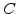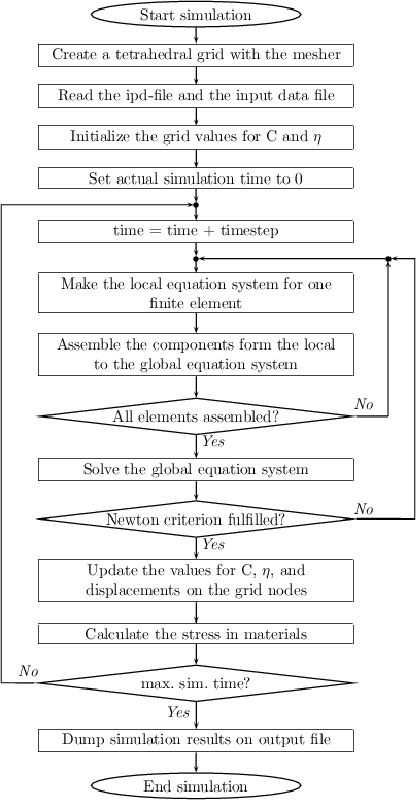Next: 6.3 Meshing Aspects Up: 6. Simulation of Thermal Previous: 6.1 Architecture of FEDOS

# 6.2 Simulation Procedure

The first step of the simulation procedure is to perform a finite element discretization by splitting up the three-dimensional structure into tetrahedral elements. A key aspect for the simulation is the number of elements, because it determines the accuracy of the simulation results and the demanded computer resources. A finer mesh with more elements means that the larger number of nodes leads to a larger equation system which needs more time for its assembling and solving procedure. The mesh generation is performed with the meshing tool which results in the input data file for FEDOS. Then the ipd-file with all the simulation parameters is imported with the Inputdeck-Reader and the file which contains the mesh, geometry, and material information is read into the WAFER-STATE-SERVER.

In the next step the initial values for the oxidant concentrationand the normalized silicon concentrationare set on the grid nodes. For examplemust be 1 in pure silicon. Because the oxidation process is time dependent, the actual oxidation time must be reset at the beginning of the simulation. As shown in Fig. 6.2, FEDOS iterates over all finite elements and builds the local equation system for every element at each actual discrete time. The local equation system describes the oxidation process numerically only for one element. In order to describe the whole oxidation process on the complete simulation domain the finite element method demands a global (coupled) equation system. The components of the global equation system are assembled from the local system by using the superposition principle as depicted in Section 5.3.1.

After the iteration over all elements is finished, the global assembled equation system, with its non-linear and linear part, is also completed. Now the global equation system can be solved with the QQQ-solver. The assembling and solving procedure is repeated, until the results from the non-linear sub-system fulfill the termination conditions of the Newton method. After the Newton system has converged the results for,, and displacements for the whole discretized oxidation process are obtained for the actual time step.

With these results the values for,, and the displacement are updated on the grid nodes such that these values are always keeping pace with the actual simulation time. The actual displacement vector enables the calculation of the strain tensor as well as the stress tensor for each element. When the above described procedure is finished, the actual simulation time is increased and the assembling for the first Newton loop is started again. The same assembling and solving procedure is repeated for each time step, until the desired end of the simulation. At the end of the simulation procedure the WAFER-STATE-SERVER writes the final simulation results to the output file.Next: 6.3 Meshing Aspects Up: 6. Simulation of Thermal Previous: 6.1 Architecture of FEDOS

Ch. Hollauer: Modeling of Thermal Oxidation and Stress Effects